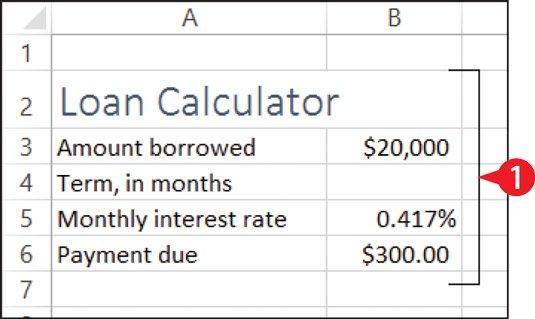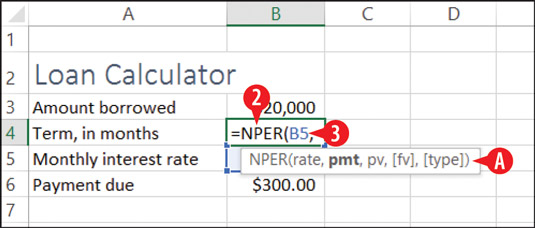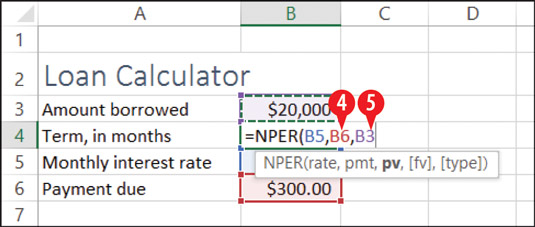##### Office 2016 at Work For DummiesOne of the most common calculation tasks in Excel is to determine the terms of a loan. There is a set of functions designed specifically for this task. Each function finds a different part of the loan equation, given the other parts:

• PV: Short for present value; finds the amount of the loan.

• NPER: Short for number of periods; finds the number of payments (the length of the loan).

• RATE: Finds the interest rate per period.

• PMT: Finds the amount of the payment per period.

Each of those functions uses the other three pieces of information as its required arguments. For example, the arguments for PV are rate, nper, and pmt.

So, let’s say, for example, that you want to know the length of a loan in which you borrow \$20,000 at 5 percent interest per year (0.417 percent per month) if you make a monthly payment of \$350. You can use the NPER function to figure that out. Here’s how:

1. In Excel, create the labels needed for the structure of the worksheet. Fill in the information you already know about the loan.Create the structure of the worksheet, including the descriptive labels and any numbers that you already know.
2. Type =NPER( into the cell where the function should be placed.

A ScreenTip reminds you of the arguments to use and their proper order.

3. Click or type the cell that contains the interest rate, and then type a comma.Begin entering the function and its arguments.
4. Click or type the cell that contains the payment amount, and then type a comma.

5. Click or type the cell that contains the loan amount, and then press Enter to complete the formula. The closing parenthesis is automatically added for you. If you do the example correctly, the loan term will show as –58.95187.Add the remaining arguments, separating them with commas.

Besides these four simple functions, there are dozens of other financial functions available in Excel. For example, IPMT is like PMT except it returns only the amount of interest in the payment, and PPMT returns only the amount of principal. Explore the functions on the Financial button’s menu on the Formulas tab on your own.

The result of the calculation will be negative if the present value (the loan amount) is a positive number. If you want the term to show as a positive number, change the amount borrowed to a negative number, or enclose the function within the ABS function (absolute value), like this: =ABS(NPER(B5,B6,B3)). ABS is short for absolute value.

Since the number of payments must be a whole number, you might choose to use the ROUNDUP function to round that value up to the nearest whole. The ROUNDUP function has two arguments: the number to be rounded and a number of decimal places. For a whole number, use 0 for the second argument. The finished formula would then look like this: =ROUNDUP(ABS(NPER(B5,B6,B3)),0).